Unlimited PowerPoint templates, graphics, videos & courses! Unlimited asset downloads! From \$16.50/m
Advertisement# Quick Start: How to Make a Basic Formula in Excel

Difficulty:BeginnerLength:MediumLanguages:
This post is part of a series called How to Make and Use Excel Formulas (Beginner Bootcamp).
How to Use the Excel VLOOKUP Function - With Useful Examples

If you've written off spreadsheets as a tool that can help you as a freelancer or creative, now's the time to rethink it. In this tutorial, I'll help you learn how to make a basic formula in Excel and get you thinking about how you can use them.

You might think of a spreadsheet as a tool for your accountant or banker, but here are ways that I use spreadsheets on a daily basis as part of my creative work:

• Planning and executing courses and tutorials that I produce for Tuts+.
• To manage other freelancers that help me run my freelance business, with shared spreadsheets in Dropbox.
• Capturing research and reference information while learning a new skill or app.

Formulas drive the usefulness of spreadsheets. Let's start learning how to use them.

A quick note: if you prefer another spreadsheet app like Apple Numbers or Google Sheets, don't stop reading. Many of the skills and formulas that we'll work with in this tutorial work in those apps as well.

## Embrace the Spreadsheet

Excel spreadsheets are a blank canvas of columns, ready for you to add data to. One of my favorite things about Excel, and spreadsheets in general, are that they scale up with your data. You can keep adding more sheets, formulas, and functions as your needs grow.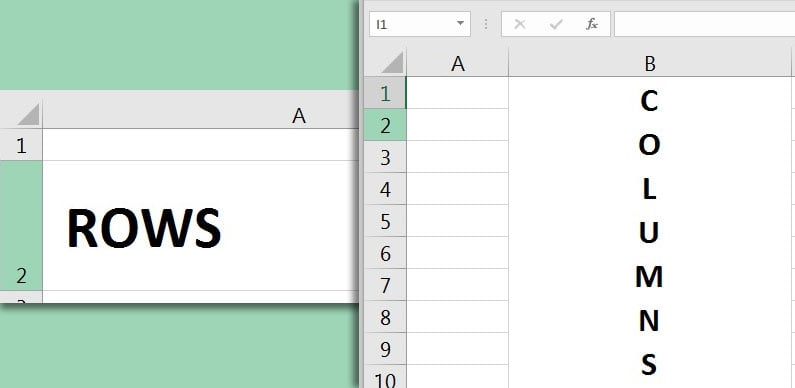Rows are the horizontal lines that are numbered, while columns are the vertical lines that are lettered.

Before we write our first formula, here are four key things to know about how spreadsheets work:

1. Spreadsheet files are usually called workbooks, with multiple tabs called sheets.
2. Excel spreadsheets are made up of rows (lines running from left to right) and columns (lines running up and down.)
3. When rows and columns meet, spreadsheets form cells.
4. Cells can hold data you type in yourself, or formulas and functions to modify and work with data.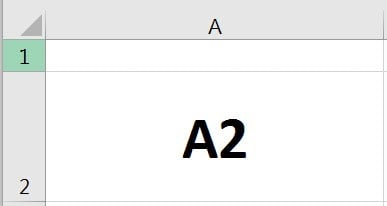When rows and columns meet, it forms cells, where we can input formulas and functions. The intersection of row 2 and column A is cell A2.

## How to Make Basic Excel Formulas & Functions (Quick Video Tutorial)

In this screencast, I'll show you the essential information for how to get started with Excel formulas, from how to make your first formula in Excel to working with basic functions.

Read on to find out about more formulas, including ten functions built into Excel to work with data.

## Your First Excel Formula

Let's write our first formula in Microsoft Excel. Write your first formula by double clicking in any Excel skill. Let's do some basic multiplication.

I'll type this into my cell:

=5*4

When I press enter, Excel will calculate the result and print 20 in the cell. The formula is still behind the scenes, and you can see it in the formula bar just above the spreadsheet.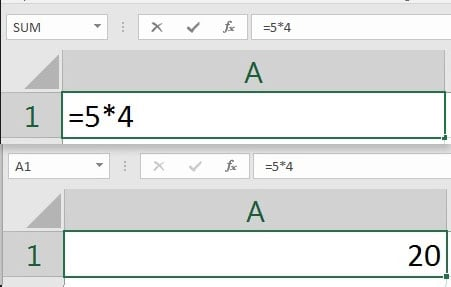This basic math formula is an example of how Excel will calculate things for us, but the formula remains behind the scenes.

This is how Excel works. Formulas operate on data, and print the output. This is an extremely basic example of how to work with data in Excel. Let's graduate to functions.

## How to Use Functions in Excel

Many people use the terms formula and function interchangeably in Microsoft Excel. In reality, the two are different:

• Formulas - Are basic operations on cells, such as using math operators like or / to add and divide numbers in your formula, for example. Formulas are simple.
• Functions - Use built-in Excel functionality, such as =AVERAGE to perform operations easily on your data. Excel comes with built-in functions that have predefined calculations you can work with, like ready-made recipes.

Most of the time, Excel users will collectively call these "formulas." Functions are actually Excel magic behind the scenes, where you can give Excel some values and Excel automates the work for you.

Functions start with an equals sign, and then the function name, and then typically an open parentheses. Then, you'll feed the function some data to work magic upon.

Let's use the AVERAGE function, for example. Instead of adding values up and dividing by the number of data, Excel automates this with the AVERAGE function. Let's look at how to use them.

To get started with the AVERAGE function, double click in an Excel cell and type =AVERAGE(, and now, we have two choices for how to average out some values:

### 1. Inline Data

You can type data right into functions in the formula. In the case of the average function, you can input values, separated by commas, and Excel will output the average of those values.

=AVERAGE(1,3,5,7,9)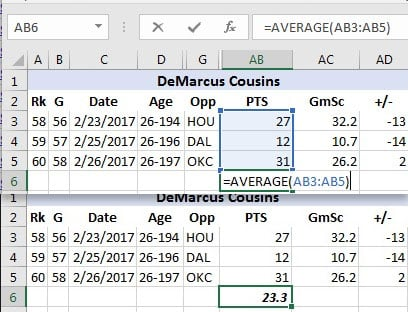Separate values with commas to average them.

### 2. Cell References

Let's say that we've already got data typed into a spreadsheet. You can use functions, and point them to other cells to average those cells instead. In the example below, I'll average a list of data with this formula:

=AVERAGE(B1:B3)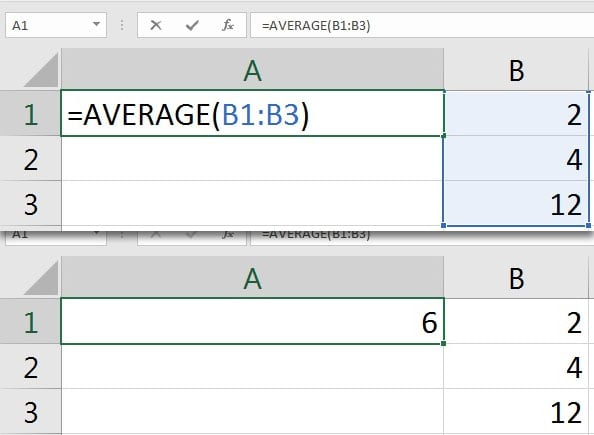Average in Excel with cell references.

The formula I'm using averages all of the data in the range that I specify, in this case cells B1 to B3.

Most functions will allow you to either work with data right inside of the formula, or run the function on data you've typed elsewhere.

## 10 Helpful Excel Formulas and Functions

So, now we've mastered how to use a basic function. The good news is that Excel offers many functions that you can use on your data. If you already know how to use one function, all others work similarly.

I spend plenty of time working in Excel every day. Here are ten formulas that I use over and over to clean up my spreadsheets or work with data, and examples of how to use them.

Note: all of these formulas work on in-line data, but would typically be used on cells you've already typed in a cell.

### 1. =AVERAGE

We've already touched on the AVERAGE function, but I think it's one of the most important ones in Excel. Whether you're averaging your grades or an NBA player's stats, this is one of the easiest and most useful formulas.

In-line usage example:

=AVERAGE(1,3,5,7,9)

Usage on cells:

=AVERAGE(A1:A5)

Example in action:

### 2. =COUNT

COUNT will help you find out how many items are in a column or a list. Count would typically only be used on a list of data, and not in-line.

Usage on cells:

=COUNT(A1:A5)

Example in action:

### 3. =UPPER, =LOWER, & =PROPER

UPPER, LOWER, and PROPER will help you clean up text that you insert into your spreadsheets. These related formulas modify text in different ways:

• UPPER - Converts a text string into all uppercase letters.
• LOWER - Converts text into all lowercase letters.
• PROPER - Converts a word into an attempted "proper" capitalization, capitalizing proper nouns.

Usage on cells:

=UPPER(A1)

=LOWER(A1)

=PROPER(A1)

Example in action:

### 4. =LEFT and =RIGHT

The LEFT and RIGHT functions will help you grab data from a part of a cell. Imagine using this formula to grab the prefix from a list of names, such as "Mr." or "Ms.", for example.

The LEFT and RIGHT formulas will grab

Usage on cells:

=LEFT(A1,5)

Gets the first five characters from cell A1.

=RIGHT(A1,5)

Gets the last five characters from cell A5.

Example in action:

### 5. =&

The =& function is used to join text and cells together. You can use it to join data from multiple cells into a single cell.

This formula works a bit differently. The formula doesn't open up with &; instead, you place it in between text or cells.

In-line usage example:

="Andrew "&"Childress"

Usage on cells:

=A1&A2

Example in action:

### 6. =MAX and =MIN

MAX and MIN are related formulas to identify the largest and smallest values in a list of data. This formula is typically used on data that you've already typed in Excel, particularly lists where you want to find the largest and smallest value in the list.

In-line usage example:

=MAX(1,3,5,7,9)

=MIN(1,3,5,7,9)

Usage on cells:

=MAX(A1:A10)

=MIN(A1:A10)

Example in action:

### 7. =TODAY

TODAY is one of the simplest and most useful functions. Simply insert it, and Excel will always keep today's date in the cell. Each time you re-open the spreadsheet, Excel will refresh the formula and put today's date in the cell.

In-line usage example:

=TODAY()

Example in action:

### 8. =TRIM

=TRIM is a function that helps you clean up your text in formulas. If your text has spaces at the beginning or end, TRIM will help to remove it.

Usage on cells:

=TRIM(A1)

Example in action:

### 9. =DAYS

=DAYS helps you calculate the number of calendar days between two dates. I like to use the DAYS formula when I'm looking at the amount of time between today and a future event, for example.

In-line usage example:

=DAYS("2/28/2016","1/1/2016")

Usage on cells:

=DAYS(B1,B2)

Example in action:

### 10. =NETWORKDAYS

=NETWORKDAYS is a function to help you calculate the number of work days between two dates. Picture yourself using this when you're calculating the number of business days left before you'll receive a package, for example.

In-line usage example:

=NETWORKDAYS("1/1/2016","2/28/2016")

Usage on cells:

=NETWORKDAYS(A2,B2)

Example in action:

These functions are powerful ways to work with data. Mastering Excel is all about combining these functions to work with data and automate your life.

## Recap and Keep Learning

If you enjoyed this tutorial on how to make a formula in Excel, and familiarized yourself with spreadsheet formulas and basic functions. I highly recommend the following resources to keep learning:

How did you get started with using spreadsheets? What do you want to learn more about? Check in with a comment to let me know.

Advertisement
Advertisement